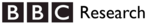Kamaelia docs : Kamaelia.Support.Data.Rationals

# Rational fraction conversion/handling

This set of functions assist in creating rational fractions (numbers represented by a fraction with an integer numerator and denominator).

## Conversion from floating point to rational fraction

The rational(...) function converts a floating point value to a rational fraction.

It aims to generate as close an approximation as is reasonably possible, and to use as small (simple) a numerator and denominator as possible.

## Examples

Conversion of a floating point number to a rational fraction:

```>>> rational(0.75)
(3, 4)
```

Scale a rational's numerator and denominator to fit within limits:

```>>> limit( (1500,2000), 80, -80)
(60, 80)
```

Find the greatest common divisor:

```>>> gcd(18,42)
6
```

## How does conversion work?

rational(...) uses the "continuous fractions" recursive approximation technique.

The algorithm effectively generates a continuous fractions up to a specified depth, and then multiplies them out to generate an integer numerator and denominator.

All depths are tried up to the maximum depth specified. The least deep one that yields an exact match is returned. This is also the simplest.

The numerator and denominator are simplified further by dividing them by their greatest common denominator.

# Feedback

Got a problem with the documentation? Something unclear that could be clearer? Want to help improve it? Constructive criticism is very welcome - especially if you can suggest a better rewording!Kamaelia is an open source project originated from and guided by BBC Research. For more information browse the site or get in contact.